1. 清华大学 水利水电工程系, 水沙科学与水利水电工程国家重点实验室, 北京 100084;
2. 清华大学 三江源协同创新中心, 北京 100084

Simulation and parameter design of laser survey in underground energy storage caverns
LÜ Xiaoning1, LIU Xiaoli1,2, DUAN Yunling1, TANG Zhili1
1. State Key Laboratory of Hydroscience and Engineering, Department of Hydraulic Engineering, Tsinghua University, Beijing 100084, China;
2. Sanjiangyuan Collaborative Innovation Center, Tsinghua University, Beijing 100084, China
Abstract: Laser survey parameters are difficult to select due to the effects of different factors on the laser measurements. The characteristics of laser survey parameters are used here to define a design criterion and a design model for the laser survey parameters. The storage cavern dimensions and application-dependent parameters were used on a laser survey simulation of an underground storage cavern based on the design model to identify the design parameters for an in-situ laser survey of an underground storage cavern. The results show that the laser survey simulation and parameter design are an efficient tool for studying the survey parameters to optimize the various tasks in the planning stage.
Key words: underground energy storage caverns     laser survey     design model     simulation     parameter design

1 激光测量参数描述

 $\begin{array}{l} {\rho _P}\left( {{\theta _P},{\alpha _P}} \right) = {\rho _P}\left( {m\Delta \theta ,n\Delta \alpha } \right) = \\ \;\;\;\;\;\sum\limits_m {\sum\limits_n {\rho \left( {m\Delta \theta ,n\Delta \alpha } \right)\delta \left( {\theta - m\Delta \theta ,\alpha - n\Delta \alpha } \right)} } . \end{array}$ (1)

1.1 激光测量设备

1.2 点云空间分辨率

 $\mathit{\Theta = }\frac{\lambda }{{{\rm{\pi }}{\omega _0}}}.$ (2)

1.3 点云间距

 ${\Delta _{{\rm{opt}}}} = 0.859\omega .$ (3)

 $\omega = 2\left( {{\omega _0} + \mathit{\Theta }\rho } \right).$ (4)

1.4 点云密度

1.5 入射角

1.6 测量参数控制标准

 测量参数 要求 测距范围/m 单站测量测点距离不能超出有效测程 测距精度/mm 满足工程要求 点云间距/mm 尽量保证点云采样最优 点云密度/(点·m-2) 尽量接近或稍大于被测对象的建模间距要求 入射角/(°) 不能超过65°，最好在45°以内

2 地下能源储库参数设计模型 2.1 地下能源储库特征

2.2 测量参数设计模型图 1 直墙圆拱洞室断面几何参数

 $\left\{ \begin{array}{l} {X_P} = {\rho _P}\cos {\theta _P}\cos {\alpha _P},\\ {Y_P} = {\rho _P}\sin {\theta _P}\cos {\alpha _P},\\ {Z_P} = h + {\rho _P}\sin {\alpha _P}. \end{array} \right.$ (5)

1) 边墙区域0＜ZPH

 ${\rho _P} = \frac{B}{{2\cos {\theta _P}\cos {\alpha _P}}}.$ (6)

 ${\varphi _P} = {\cos ^{ - 1}}\left( {\cos {\theta _P}\cos {\alpha _P}} \right).$ (7)

2) 底板区域ZP=0。

 ${\rho _P} = \frac{{ - h}}{{\sin {\alpha _P}}}.$ (8)

 ${\varphi _P} = {\cos ^{ - 1}}\left( { - \sin {\alpha _P}} \right).$ (9)

3) 拱顶区域ZPH

 $\begin{array}{l} {\rho _P} = \frac{{ - b + \sqrt {{b^2} - 4ac} }}{{2a}},\\ a = 4H_a^2{\cos ^2}{\theta _P}{\cos ^2}{\alpha _P} + {B^2}{\sin ^2}{\alpha _P},\\ b = 2{B^2}\left( {h - H} \right)\sin {\alpha _P},\\ c = {B^2}{\left( {h - H} \right)^2} - {B^2}H_a^2. \end{array}$ (10)

 $\begin{array}{l} {\varphi _P} = {\cos ^{ - 1}}\left( {\frac{{2a{\rho _P} + b}}{{2\sqrt {d\rho _P^2 + {B^2}\left( {b{\rho _P} + e} \right)} }}} \right),\\ d = 16h_a^4{\cos ^2}{\theta _P}{\cos ^2}{\alpha _P} + {B^4}{\sin ^2}{\alpha _P},\\ e = {B^2}{\left( {h - H} \right)^2}. \end{array}$ (11)

 $\begin{array}{l} {\omega _a} = \frac{{2\left( {{\omega _0} + \mathit{\Theta }{\rho _P}} \right)}}{{\cos {\varphi _P}}},\\ {\omega _b} = 2{\omega _0} + \mathit{\Theta }{\rho _P}. \end{array}$ (12)

 $\begin{array}{l} {\Delta _{\rm{H}}} = \frac{{{\rho _P}\Delta \theta }}{{\cos {\varphi _P}}} = \frac{{{\rm{FO}}{{\rm{V}}_{\rm{H}}}}}{{{\rm{PP}}{{\rm{R}}_{\rm{H}}}\cos {\varphi _P}}}{\rho _P},\\ {\Delta _{\rm{V}}} = \frac{{{\rho _P}\Delta \alpha }}{{\cos {\varphi _P}}} = \frac{{{\rm{FO}}{{\rm{V}}_{\rm{V}}}}}{{{\rm{PP}}{{\rm{R}}_{\rm{V}}}\cos {\varphi _P}}}{\rho _P},\\ {\rm{PD = }}\frac{1}{{{\Delta _{\rm{H}}}{\Delta _{\rm{V}}}}}. \end{array}$ (13)

 $\begin{array}{l} {\Delta _{\rm{H}}} = \frac{v}{{{\rm{LPS}}}},\\ {\Delta _{\rm{V}}} = \frac{{{\rm{FO}}{{\rm{V}}_{\rm{V}}} \cdot {\rm{LPS}}}}{{{\rm{PPR}}}}{\rho _P},\\ {\rm{PD = }}\frac{1}{{{\Delta _{\rm{H}}}{\Delta _{\rm{V}}}}}. \end{array}$ (14)
2.3 参数设计流程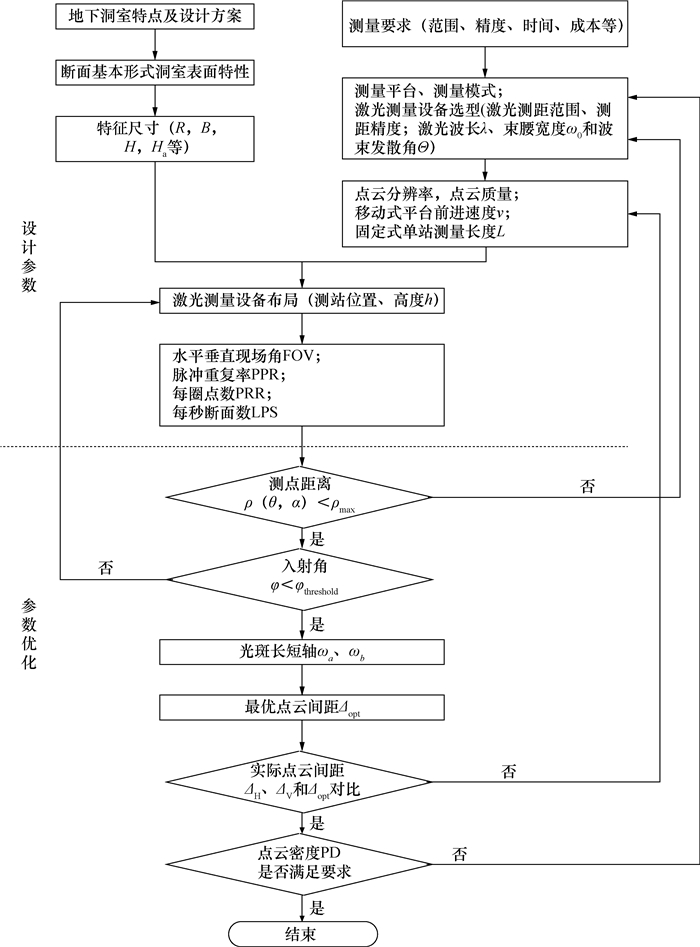图 2 激光测量参数设计流程

3 工程实例验证 3.1 基本资料

3.2 固定式激光测量模拟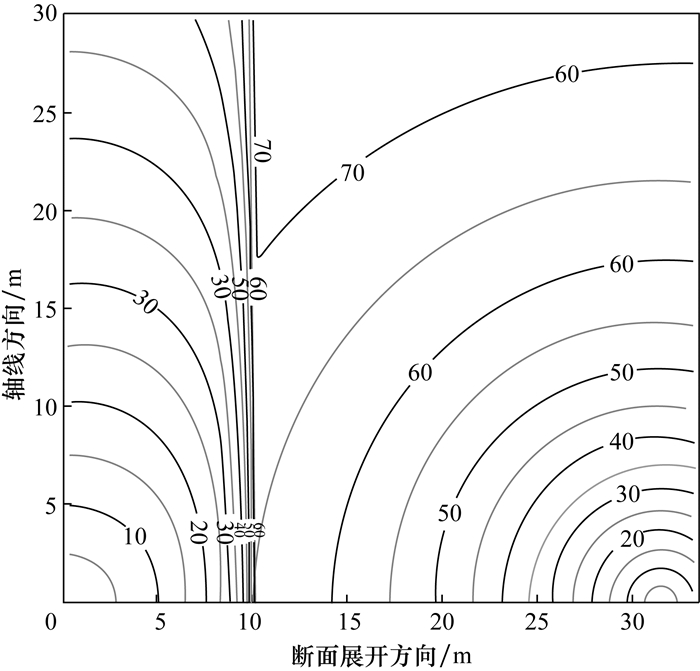图 3 固定式激光测量入射角空间分布图(单位：(°))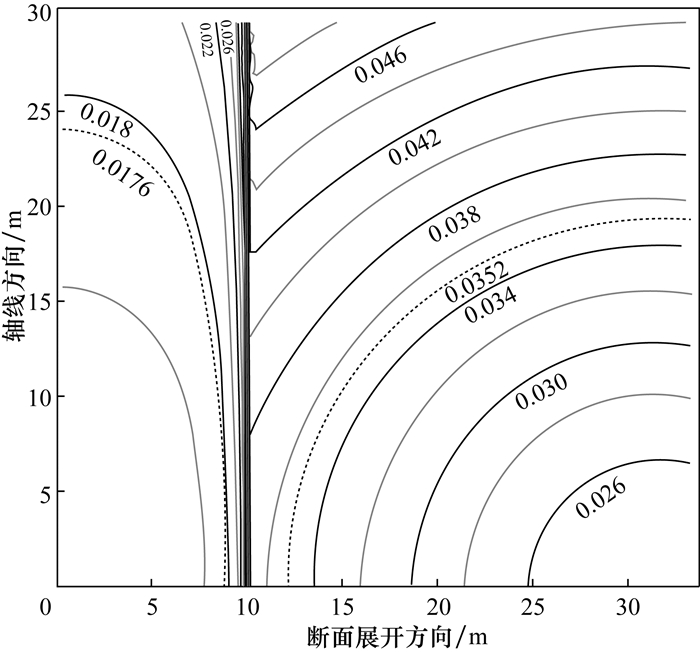图 4 固定式激光测量最优角度分辨率空间分布图(单位：(°))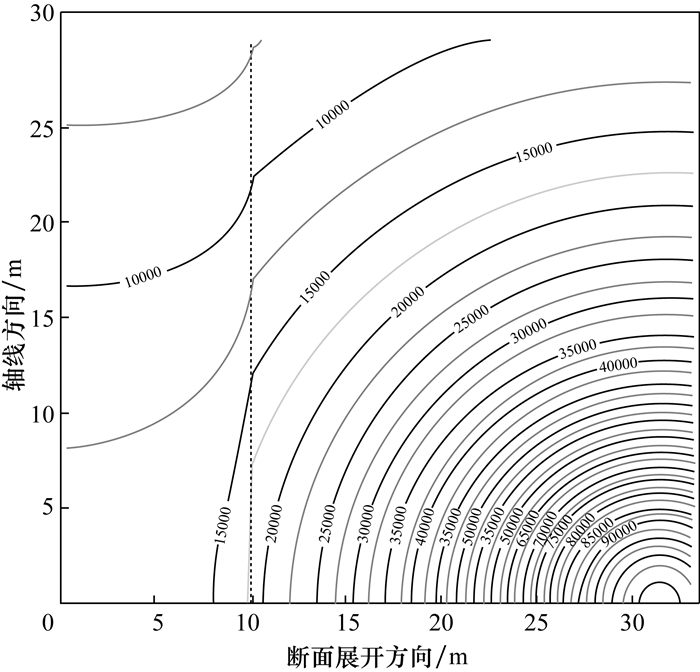图 5 固定式激光测量分辨率为1/2点云密度空间分布图(单位：点·m-2)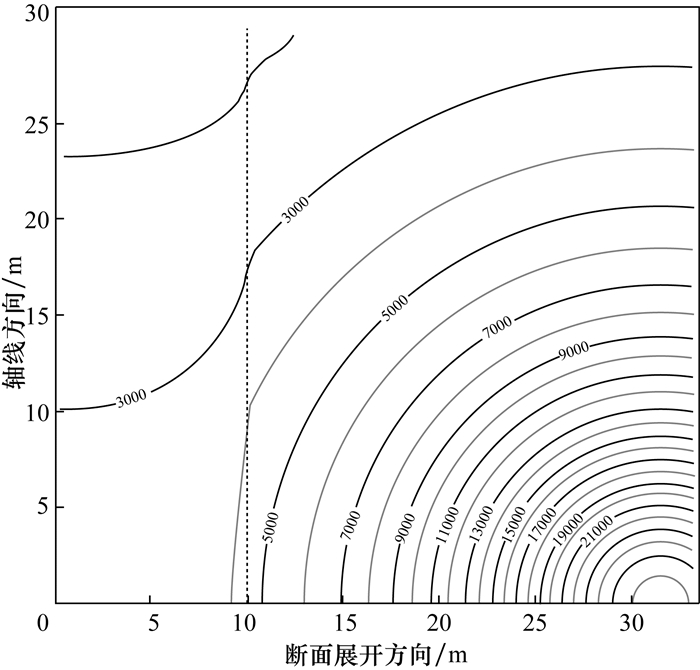图 6 固定式激光测量分辨率为1/4点云密度空间分布图(单位：点·m-2)

3.3 移动式激光测量模拟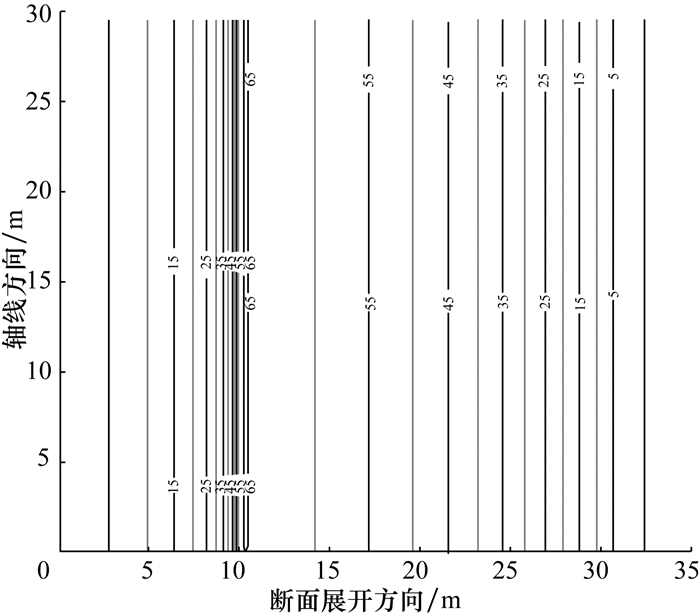图 7 移动式激光测量入射角空间分布图(单位：(°))图 8 移动式激光测量最优角度分辨率空间分布图(单位：(°))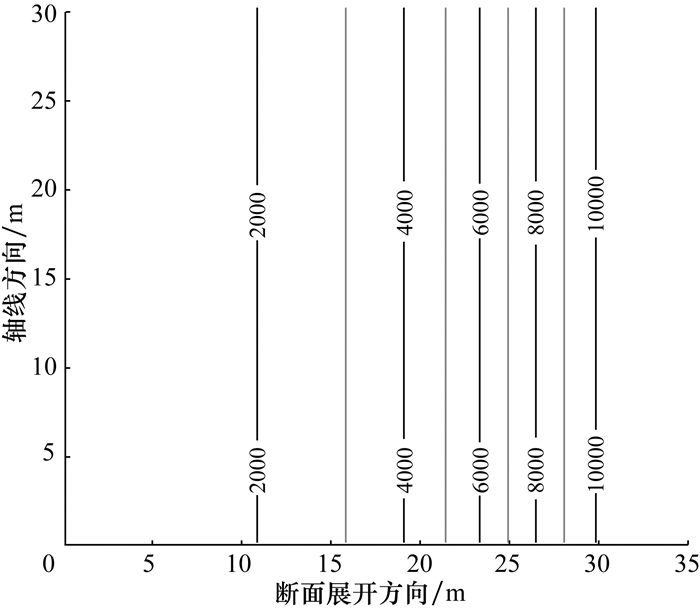图 9 移动式激光测量分辨率为1/2点云密度空间分布图(单位：点·m-2)

3.4 现场试验图 10 移动式激光测量点云三维效果图

4 结论

  Soudarissanane S, Lindenbergh R, Menenti M, et al. Scanning geometry: Influencing factor on the quality of terrestrial laser scanning points[J]. ISPRS J Photogramm Remote Sens, 2011, 66(4): 389–399. DOI:10.1016/j.isprsjprs.2011.01.005  Kukko A, Hyyppa J. Small-footprint laser scanning simulator for system validation, error assessment, and algorithm development[J]. Photogrammetric Engineering & Remote Sensing, 2009, 75(9): 1177–1189.  Pesci A, Teza G, Bonali E. Terrestrial laser scanner resolution: Numerical simulations and experiments on spatial sampling optimization[J]. Remote Sensing, 2011, 3(1): 167–184.  Arguelles-Fraga R, Ordonez C, Garcia-Cortes S, et al. Measurement planning for circular cross-section tunnels using terrestrial laser scanning[J]. Automat Constr, 2013, 31(3): 1–9.  Roca-Pardinas J, Arguelles-Fraga R, de Asis Lopez F, et al. Analysis of the influence of range and angle of incidence of terrestrial laser scanning measurements on tunnel inspection[J]. Tunn Undergr Sp Tech, 2014, 43(7): 133–139.  Buckley S J, Howell J A, Enge H D, et al. Terrestrial laser scanning in geology: Data acquisition, processing and accuracy considerations[J]. Journal of the Geological Society, 2008, 165(3): 625–638. DOI:10.1144/0016-76492007-100  Gikas V. Three-dimensional laser scanning for geometry documentation and construction management of highway tunnels during excavation[J]. Sensors, 2012, 12(8): 11249–11270.  Lichti D D, Jamtsho S. Angular resolution of terrestrial laser scanners[J]. The Photogrammetric Record, 2006, 21(114): 141–160. DOI:10.1111/phor.2006.21.issue-114  MacEachren A M, Davidson J V. Sampling and isometric mapping of continuous geographic surfaces[J]. The American Cartographer, 1987, 14(4): 299–320. DOI:10.1559/152304087783875723  Lichti D D. Error modelling, calibration and analysis of an AM-CW terrestrial laser scanner system[J]. ISPRS J Photogramm Remote Sens, 2007, 61(5): 307–324. DOI:10.1016/j.isprsjprs.2006.10.004  Delaloye D, Diederichs M S, Walton G, et al. Sensitivity testing of the newly developed elliptical fitting method for the measurement of convergence in tunnels and shafts[J]. Rock Mech Rock Eng, 2015, 48(2): 651–667. DOI:10.1007/s00603-014-0566-0  Zhao J, Choa V, Broms B B. Construction and utilization of rock caverns in Singapore Part B: Development costs and utilization[J]. Tunn Undergr Sp Tech, 1996, 11(1): 73–79. DOI:10.1016/0886-7798(96)00055-7  Lee C I, Song J J. Rock engineering in underground energy storage in Korea[J]. Tunn Undergr Sp Tech, 2003, 18(5): 467–483. DOI:10.1016/S0886-7798(03)00046-4  何世德. 三心拱弧与椭圆弧[J]. 河北煤炭, 1987(4): 15–18. HE Shide. Three-centered circular arch and elliptical arch[J]. Hebei Coal, 1987(4): 15–18. (in Chinese)  Trimble. Trimble TX5 scanner datasheet [EB/OL]. (2012-11-07). http://www.trimble.com.